Author: Oscar Cronquist Article last updated on December 23, 2018

### Match a date when a date range is entered in a single cell

Column C contains the start and end date separated by a forward slash /. The formula in cell C9 splits the dates and checks if the date in cell C8 is in a date range and if it is it returns the adjacent value on the same row.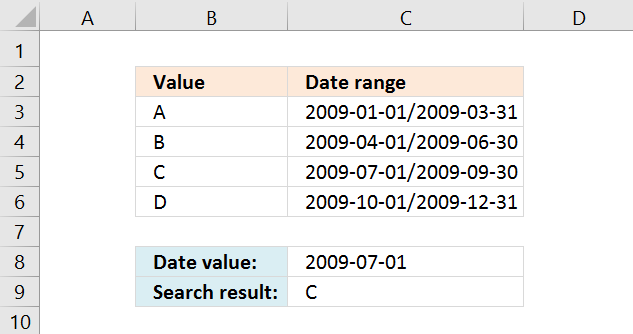Formula in C9:

=INDEX(\$B\$3:\$B\$6, SUMPRODUCT(DATEVALUE(RIGHT(C3:C6, LEN(C3:C6)-FIND("/", C3:C6)))>=C8)*(DATEVALUE(LEFT(C3:C6, FIND("/", C3:C6)-1))<=C8), MATCH(ROW(\$C\$3:\$C\$6), ROW(\$C\$3:\$C\$6))))

#### Watch a video where I explain the formula above

Recommended articles

Find date range based on a date

This article demonstrates a formula that returns a date range that a date falls under, cell C3 above contains the […]

How to return a value if lookup value is in a range

In this article, I will demonstrate four different formulas that allow you to lookup a value that is to be found […]

#### How to create an array formula

1. Select cell C9
2. Press with left mouse button on in formula bar
3. Copy and paste array formula to formula bar
4. Press and hold CTRL + SHIFT
5. Press Enter
6. Release all keys

Recommended article

A beginners guide to Excel array formulas

Array formulas allows you to do advanced calculations not possible with regular formulas.

#### Get excel file, see sheet Ex 1

matching-a-date-in-a-date-range v3.xlsx
(Excel 2007- Workbook *.xlsx)

### Match a date when a date range is entered in two cells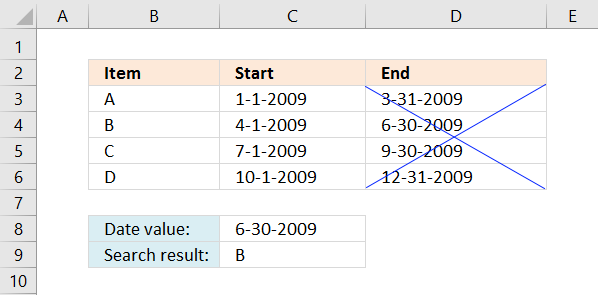There are no gaps between these date ranges which makes it is possible to only use the dates in column C in the following formula:

Formula in cell C9:

=LOOKUP(C8,C3:C6,B3:B6)

#### Watch a video where I explain the formula above

Remember, the LOOKUP function requires date values to be sorted in an ascending order in column C. If not, use the formula below.

If you do have gaps between some date ranges, like the picture below. There is a date gap between 3-1-2009 and 4-1-2009. You then need to use both the start and end date to find the value you are looking for.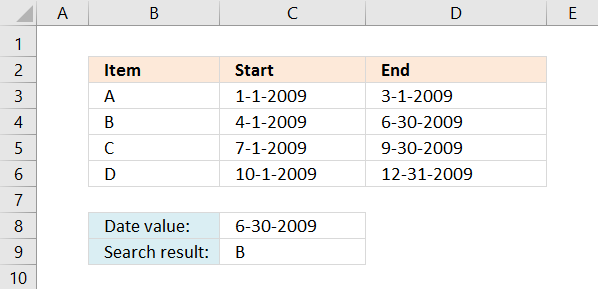Formula in cell C9:

=INDEX(\$B\$3:\$B\$6,SUMPRODUCT((\$C\$8>=\$C\$3:\$C\$6)*(\$C\$8<=\$D\$3:\$D\$6)*MATCH(ROW(\$B\$3:\$B\$6),ROW(\$B\$3:\$B\$6))))

If the user enters a value outside the date ranges an error #N/A is returned.

#### Watch a video where I explain the formula above

Recommended article

Calculate date given weekday and week number

This article demonstrates a formula that returns a date based on a week number and a weekday like Sun to […]

#### How to enter an array formula

1. Select cell C9
2. Paste formula
3. Press and hold Ctrl + Shift
4. Press Enter

#### Explaining formula in cell C9

Step 1 - Check if date is in any of the date ranges

(\$C\$8>=\$C\$3:\$C\$6)*(\$C\$8<=\$D\$3:\$D\$6)

becomes

(39994>={39814;39904;39995;40087})*(39994<={39903;39994;40086;40178})

becomes

{TRUE;TRUE;FALSE;FALSE}*{FALSE;TRUE;TRUE;TRUE}

and returns

{0;1;0;0}

Step 2 - Multiply with relative row number

(\$C\$8>=\$C\$3:\$C\$6)*(\$C\$8<=\$D\$3:\$D\$6)* MATCH(ROW(\$B\$3:\$B\$6), ROW(\$B\$3:\$B\$6))

becomes

{0;1;0;0}*MATCH(ROW(\$B\$3:\$B\$6), ROW(\$B\$3:\$B\$6))

becomes

{0;1;0;0}*{1;2;3;4}

and returns {0;2;0;0}

Step 3 - Sum values in array

SUMPRODUCT((\$C\$8>=\$C\$3:\$C\$6)*(\$C\$8<=\$D\$3:\$D\$6)* MATCH(ROW(\$B\$3:\$B\$6), ROW(\$B\$3:\$B\$6)))

becomes

SUMPRODUCT({0;2;0;0})

and returns 2.

Step 4 - Return value from cell range

INDEX(\$B\$3:\$B\$6, SUMPRODUCT((\$C\$8>=\$C\$3:\$C\$6)*(\$C\$8<=\$D\$3:\$D\$6)* MATCH(ROW(\$B\$3:\$B\$6), ROW(\$B\$3:\$B\$6))))

becomes

INDEX(\$B\$3:\$B\$6, 2)

becomes

INDEX({"A";"B";"C";"D"}, 2)

and returns B in cell C9.

### Get the Excel filematching-a-date-in-a-date-range-v3.xlsx

### Use VLOOKUP to search date in date ranges and return value on the same row

The following formula uses only the VLOOKUP function, however, the dates must be sorted in an ascending order and if a date is outside a date ranges 0 (zero) is returned. There can't be any overlapping date ranges and the formula can only return one value.

The example below has date ranges in only one column.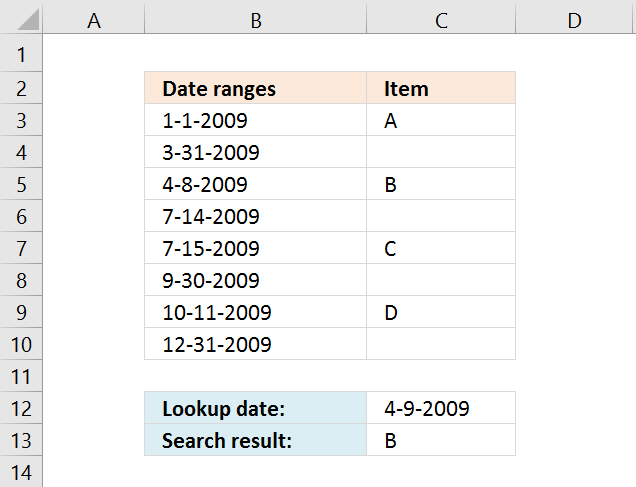You are also required to have the lookup column in the first column in the cell reference you use in the VLOOKUP function. Example, the second argument in the VLOOKUP function below is this cell reference: B3:C10. The lookup column must be in column B.

Formula in cell C13:

=VLOOKUP(C12,B3:C10,2,TRUE)

Important! The first date range seems to be 1-1-2009/3-31-2009 but it is actually 1-1-2009/3-30-2009, why is this? The VLOOKUP function matches the largest date that is smaller or equal to the lookup date. If the lookup date is 3-31-2009 it will match 3-31-2009 found in cell B4 and return the corresponding value in column C (cell C4). In this case nothing, cell C4 is empty. This applies to all date ranges in column B.

You need to change your date ranges accordingly if you want to use the VLOOKUP function for date ranges entered vertically. However, the VLOOKUP function works perfectly fine if you have date ranges with no gaps between the end dates and start dates, see picture below. You then only need to use the start dates for each date range, example demonstrated in column C see picture below.Recommended article

How to return a value if lookup value is in a range

In this article, I will demonstrate four different formulas that allow you to lookup a value that is to be found […]

#### Get excel file, see sheet Ex 4

matching-a-date-in-a-date-range v3.xlsx
(Excel 2007- Workbook *.xlsx)

### Match a date when date ranges sometimes overlap and return multiple results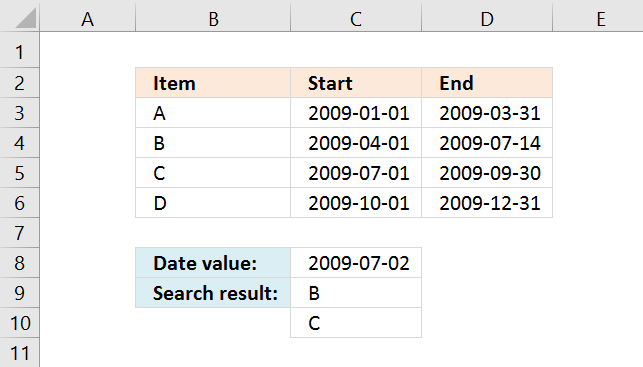Array formula in cell C9:

=INDEX(\$B\$3:\$B\$6, SMALL(IF((\$C\$8>=\$C\$3:\$C\$6)*(\$C\$8<=\$D\$3:\$D\$6), MATCH(ROW(\$B\$3:\$B\$6), ROW(\$B\$3:\$B\$6))), ROW(A1)))

Recommended article

Identify overlapping date ranges

The formula in cell F6 returns TRUE if the date range on the same row overlaps another date range in […]

#### How to enter an array formula

1. Select cell C9
2. Paste formula
3. Press and hold Ctrl + Shift
4. Press Enter

#### How to copy array formula

1. Select cell C9
2. Copy cell (not formula)
3. Select cell range C10:C11
4. Paste

#### Get excel file, see sheet Ex 3

matching-a-date-in-a-date-range v3.xlsx
(Excel 2007- Workbook *.xlsx)

SUMPRODUCT(array1, array2, )
Returns the sum of the products of the corresponding ranges or arrays

FIND(find_text,within_text,[start_num])
Returns the starting position of one text string within another text string. FIND is case-sensitive

INDEX(array,row_num,[column_num])
Returns a value or reference of the cell at the intersection of a particular row and column, in a given range

LEFT(text,num_chars)
Returns the specified number of characters from the start of textstring

RIGHT(text,num_chars)
Returns the specified number of characters from the end of textstring

ROW(reference)
Returns the rownumber of a reference

LEN(text)
Returns the number of characters in a text string

DATEVALUE(date_text)
Converts a date in the form of text to a number that represents the date in Microsoft Office Excel date-time code